Starting Mathematica

This is a basic introduction to Mathematica. Unlike conventional computer languages Mathematica can do symbolic manipulation, has a huge number of built-in numerical functions, can do numerical calculations to arbitrary precision, and has powerful plotting routines which interface directly with the results of calculations. Mathematica can also be used for programming in styles which are quite different from those of C and fortran, but we will not discuss programming in Mathematica here.
There are two interfaces to Mathematica: (i) a command line interface, and (ii) the notebook interface. The same commands are given in both, but the notebook interface has some additional features.

If one starts Mathematica in Windows  by clicking on an icon one goes straight into the notebook interface in which a separate window appears into which Mathematica commands are typed.  To execute a command in Windows, you need to hold down the Shift key as well as pressing Enter (just pressing Enter allows you to continue entering your command on the next line but does not execute it).

A Simple Mathematica Session

Mathematica understands the usual operators + - * / and for exponentiation. It also understands basic constants pi and E.  It also knows about a large number of mathematical functions such as Exp[x], Sin[x], Cos[x], ArcSin[x], Log[x]

The following session illustrates some of these features:

x = 7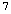y = 19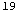x y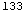(x + 3) y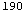2^x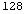Exp[ Pi]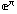Two-Dimensional Plots

The basic plotting command is Plot[f, {x, xmin, xmax}]
which plots the function f(x) from xmin to xmax
For example,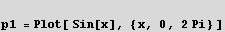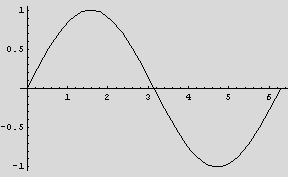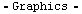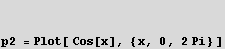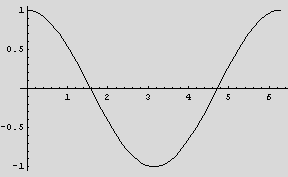The command Show can also be used to combine plots. Suppose we create plots of two functions using two separate calls to Plot, e.g.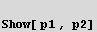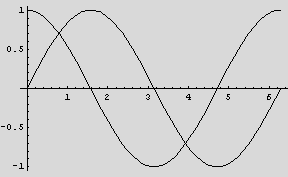Three-Dimensional Plots

We will learn how to plot cool surfaces like this one...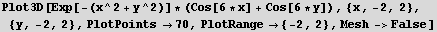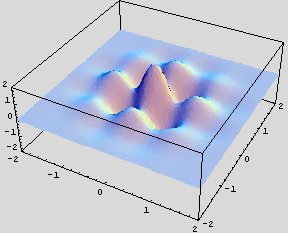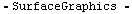We will also learn how to plot curves in three dimensions.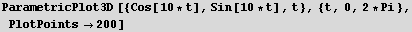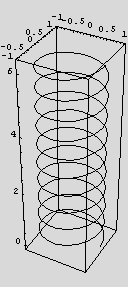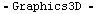Integration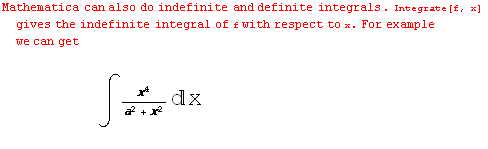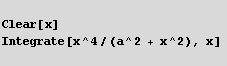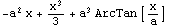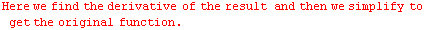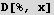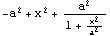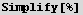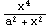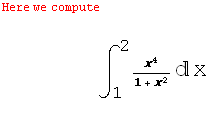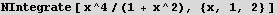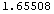Defining Functions

There are many functions in Mathematica but it is often very useful to be able to define functions oneself. This is illustrated by the following example, which defines the function . f(x) = x + x^2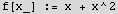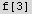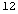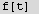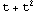Note two things about the first line:. First of all the underscore after the x, which is called a ``blank'', stands for ``any expression'', so when the function is called, the argument can be named anything, not necessarily x. Secondly, the use of :=, rather than =, which means that the assignment is delayed until the function is called. In the present example it wouldn't have made any difference if we had used =, the normal assignment operator, but it would if the function depended on a parameter.

Series Expansions
.
Mathematica can work out series of complicated functions to very high order. The command is Series[f, {x, x0, n}] which expands f in powers of up to n-th order, e.g. to get the first 20 terms in the expansion of about x=0: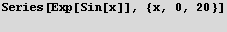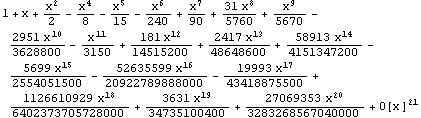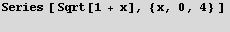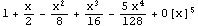Solving Differential Equations

DSolve[eqn, y, x] solves a differential equation for the function y, with independent variable x.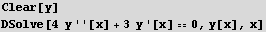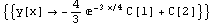Here we solve Cauchy-Euler equation.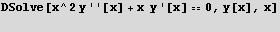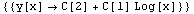Eigenvalues & Matrices

Eigenvalues[m] gives a list of the eigenvalues of the square matrix m.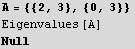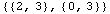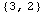Here we find eigenvectors.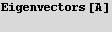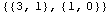Here we find CharacteristicPolynomial.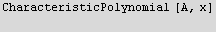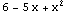Here we find the determinant.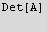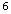Here we find the inverse.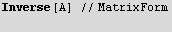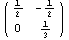Here we use row operations to reduce the matrix.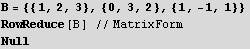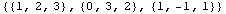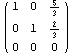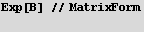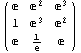Laplace Transform

LaplaceTransform[Sin[t],t,s]

Series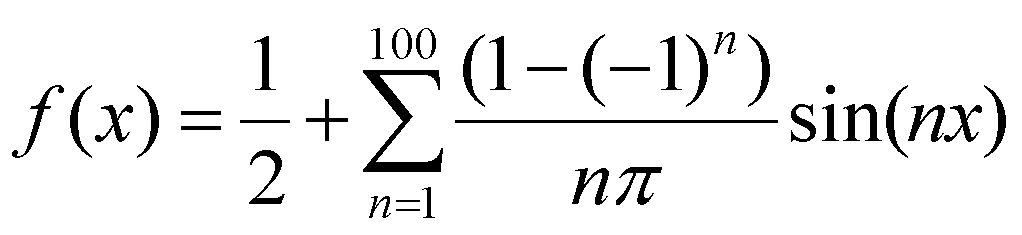0.5+Sum[ (1-(-1)^n)*Sin[n x]/(n Pi),{n,1,100}]

To plot f(x)

Plot[0.5+Sum[ (1-(-1)^n)*Sin[n x]/(n Pi),{n,1,1000}],{x,-Pi,Pi}];

Created by Mathematica  (May 16, 2006)